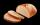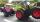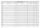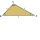# Reason - examples - page 31

1. Digit sumThe digit sum of the two-digit number is nine. When we turn figures and multiply by the original two-digit number, we get the number 2430. What is the original two-digit number?
2. BreadsSeveral pieces of bread were in the store. Half of them plus a half of bread bought a cookbook. Of the remaining bread, half of it plus half of the bread was bought by Mr. Novák. The last bread was bought by Ms. Small. How many breads were in the store at.
3. Ľé sweetsThere are 20 sweets in the bag. Some are chocolate, other coconuts, and the remaining marzipan. Chocolate is 4 times more than coconut. Marzipan's less than chocolate. How much is in a bag of coconut sweets?
4. CloudsApproximately at what height is the cloud we see under an angle of 26°10' and see the Sun at an angle of 29°15' and the shade of the cloud is 92 meters away from us?
5. Train delayThe train should be in the station for 10 minutes. It still has 32 km left. What is the expected delay if the train travels in 2 minutes 3 km except for the last section where it is 2 km in 5 minutes.
6. ProveProve that k1 and k2 is the equations of two circles. Find the equation of the line that passes through the centers of these circles. k1: x2+y2+2x+4y+1=0 k2: x2+y2-8x+6y+9=0
7. ThalesThales is 1 m from the hole. The eyes are 150 cm above the ground and look into the hole with a diameter of 120 cm as shown. Calculate the depth of the hole.
8. Three friendsThree friends had balls in ratio 2: 7: 4 at the start of the game. Could they have the same number of balls at the end of the game? Write 0, if not, or write the minimum number of balls they had together.
9. DiceWe throw five times the dice. What is the probability that six fits exactly twice?
10. Book readingIf we read the book at a speed of 15 pages a day, we read it 3 days before we read it at a speed of 10 pages per day. If I read at 6 pages per day, how many days will I read the book?
11. Basket of fruitIn six baskets, the seller has fruit. In individual baskets, there are only apples or just pears with the following number of fruits: 5,6,12,14,23 and 29. "If I sell this basket," the salesman thinks, "then I will have just as many apples as a pear." Which
12. Repair pipe20 workers had to repair broken pipes in 30 days. After fourteen days, four laborers joined them. How long did the pipe repair work last?
13. ApplesSchool kitchen bought 36kg apples for 12 kc/kg. How many kilograms of apples 1/4 cheaper can they buy for the same money?
14. TractorsTwo tractors plow the field in 4 hours together. If the first tractor plow half of the field and then the second tractor completed the job, it would take 9 hours. How many hours does the field plow for each tractor separately?
15. ABCD squareIn the ABCD square, the X point lies on the diagonal AC. The length of the XC is three times the length of the AX segment. Point S is the center of the AB side. The length of the AB side is 1 cm. What is the length of the XS segment?
16. Quarter circleWhat is the radius of a circle inscribed in the quarter circle with a radius of 100 cm?
17. Equilateral triangleA square is inscribed into an equilateral triangle with a side of 10 cm. Calculate the length of the square side.
18. Class alphabetAll 29 pupils in the class are written in a class by alphabet. The number of pupils enrolled before Paul is three times higher than the number of pupils behind him. Calculate how many pupils are enrolled after Paul.
19. Endless lego setThe endless lego set contains only 6, 9, 20 kilograms blocks that can no longer be polished or broken. The workers took them to the gym and immediately started building different buildings. And of course, they wrote down how much the building weighed. They
20. TrianglesFive sticks with a length of 2,3,4,5,6 cm. How many ways can you choose three sticks to form three sides of a triangle?

Do you have an interesting mathematical example that you can't solve it? Enter it, and we can try to solve it.

To this e-mail address, we will reply solution; solved examples are also published here. Please enter e-mail correctly and check whether you don't have a full mailbox.